# Learning Grid-like Units with Vector Representation of Self-Position and Matrix Representation of Self-Motion

Ruiqi Gao 1*, Jianwen Xie 2*, Song-Chun Zhu 1, and Ying Nian Wu 1

(* Equal contributions)
1 University of California, Los Angeles (UCLA), USA
2 Hikvision Research Institute, Santa Clara, USA

## Abstract

This paper proposes a representational model for grid cells. In this model, the 2D self-position of the agent is represented by a high-dimensional vector, and the 2D self-motion or displacement of the agent is represented by a matrix that transforms the vector. Each component of the vector is a unit or a cell. The model consists of the following three sub-models. (1) Vector-matrix multiplication. The movement from the current position to the next position is modeled by matrix-vector multiplication, i.e., the vector of the next position is obtained by multiplying the matrix of the motion to the vector of the current position. (2) Magnified local isometry. The angle between two nearby vectors equals the Euclidean distance between the two corresponding positions multiplied by a magnifying factor. (3) Global adjacency kernel. The inner product between two vectors measures the adjacency between the two corresponding positions, which is defined by a kernel function of the Euclidean distance between the two positions. Our representational model has explicit algebra and geometry. It can learn hexagon patterns of grid cells, and it is capable of error correction, path integral and path planning.

## Code

If you wish to use our code or results, please cite the following paper:

Learning Grid-like Units with Vector Representation of Self-Position and Matrix Representation of Self-Motion
@article{gao2018learning, title={Learning Grid-like Units with Vector Representation of Self-Position and Matrix Representation of Self-Motion}, author={Gao, Ruiqi and Xie, Jianwen and Zhu, Song-Chun and Wu, Ying Nian}, journal={arXiv preprint arXiv:1810.05597}, year={2018}}

## Background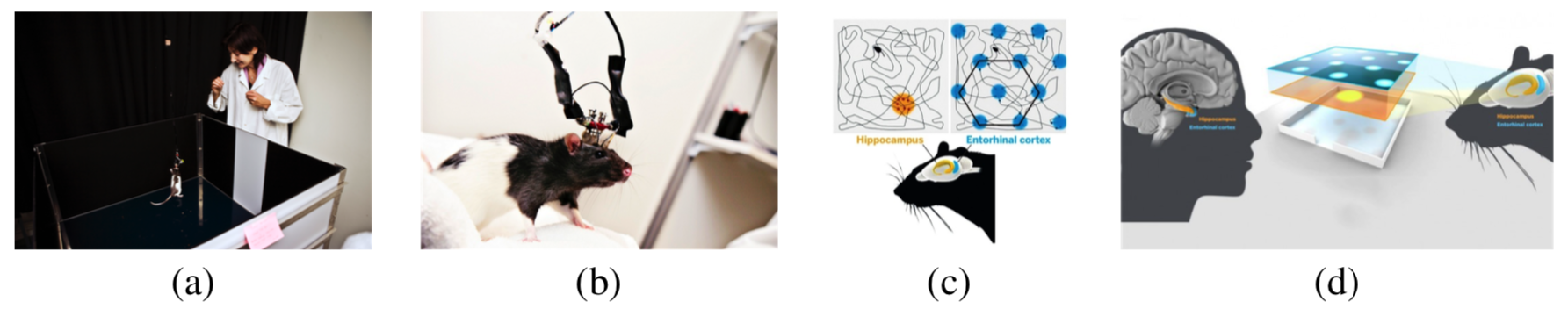Figure 1. Place cells and grid cells. (a) The rat is moving within a square region. (b) The activity of a neuron is recorded. (c) When the rat moves around (the curve is the trajectory), each place cell fires at a particular location, but each grid cell fires at multiple locations that form a hexagon grid. (d) The place cells and grid cells exist in the brains of both rat and human. (Source of pictures: internet)

## Model illustrations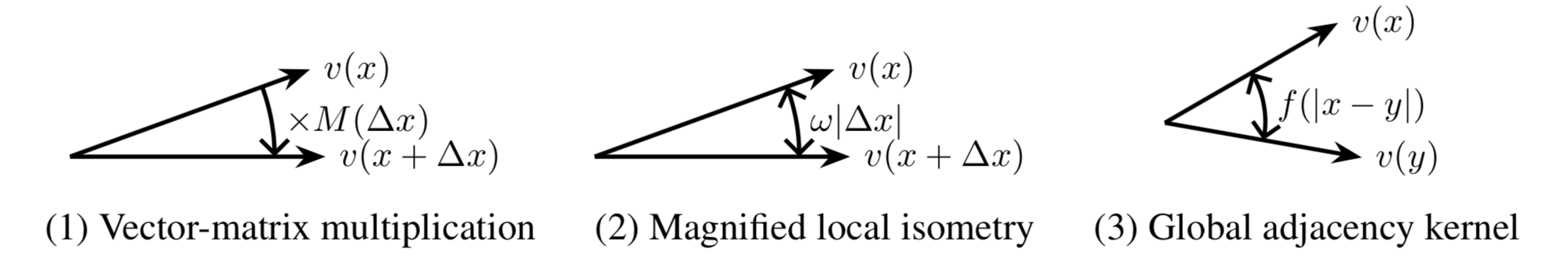Figure 2. Grid cells form a high-dimensional vector representation of 2D self-position. Three sub- models: (1) Local motion is modeled by vector-matrix multiplication. (2) Angle between two nearby vectors magnifies the Euclidean distance. (3) Inner product between any two vectors measures the adjacency which is a kernel function of the Euclidean distance.

## Experiments

Contents

Exp 1 : Learning Single Blocks: Hexagon Patterns and Metrics
Exp 2 : Learning Multiple Hexagon Blocks and Metrics
Exp 3 : Path Integral
Exp 4 : Path Planning
Exp 5 : Quantitative analysis of spatial activity
Exp 6 : Error correction

### Experiment 1: Learning Single Blocks: Hexagon Patterns and Metrics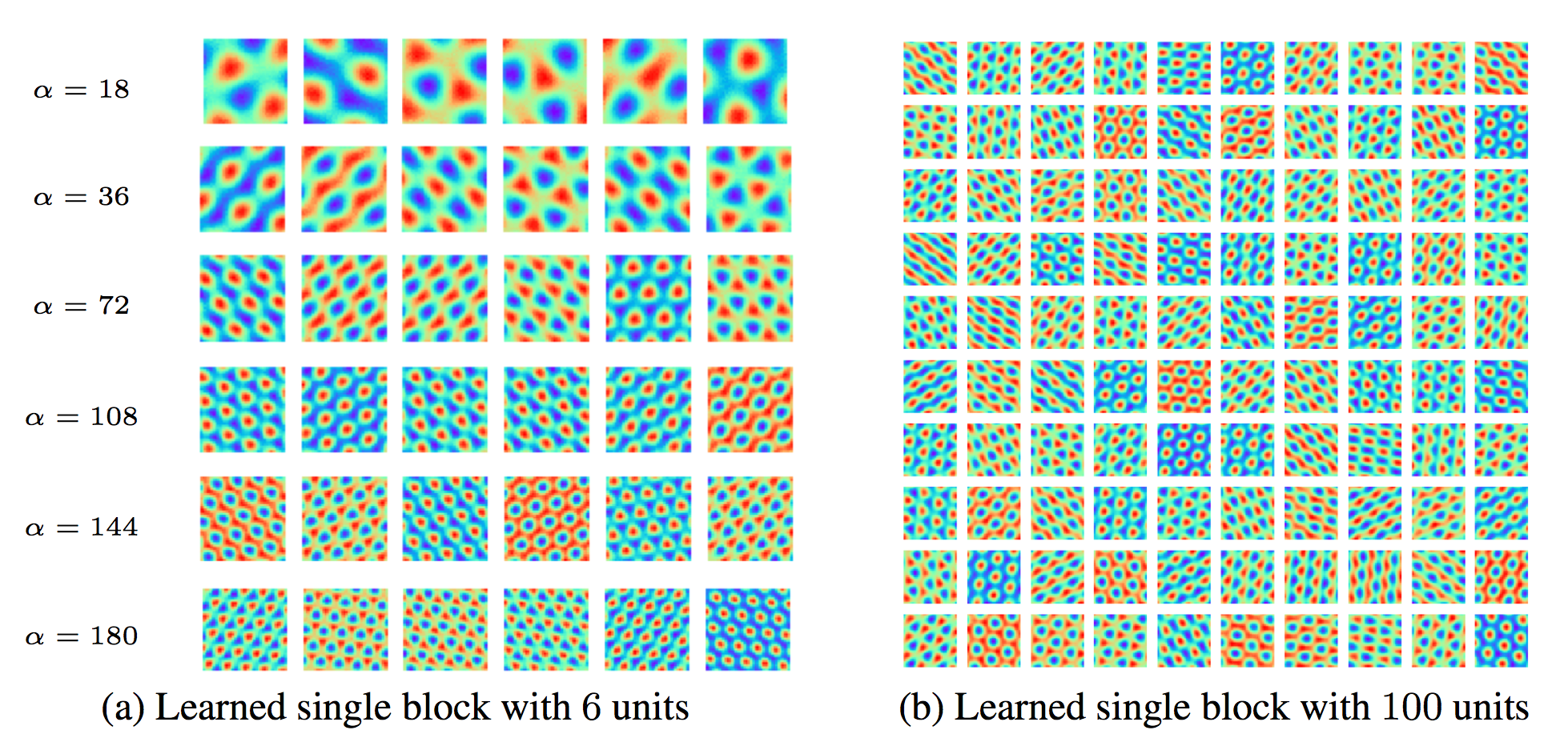Figure 3. Learned units of the vector representation using single block. (a) Learned single block with 6 units. Every row shows the learned units with a certain α. (b) Learned single block with 100 units and α = 72.

### Experiment 2: Learning Multiple Hexagon Blocks and Metrics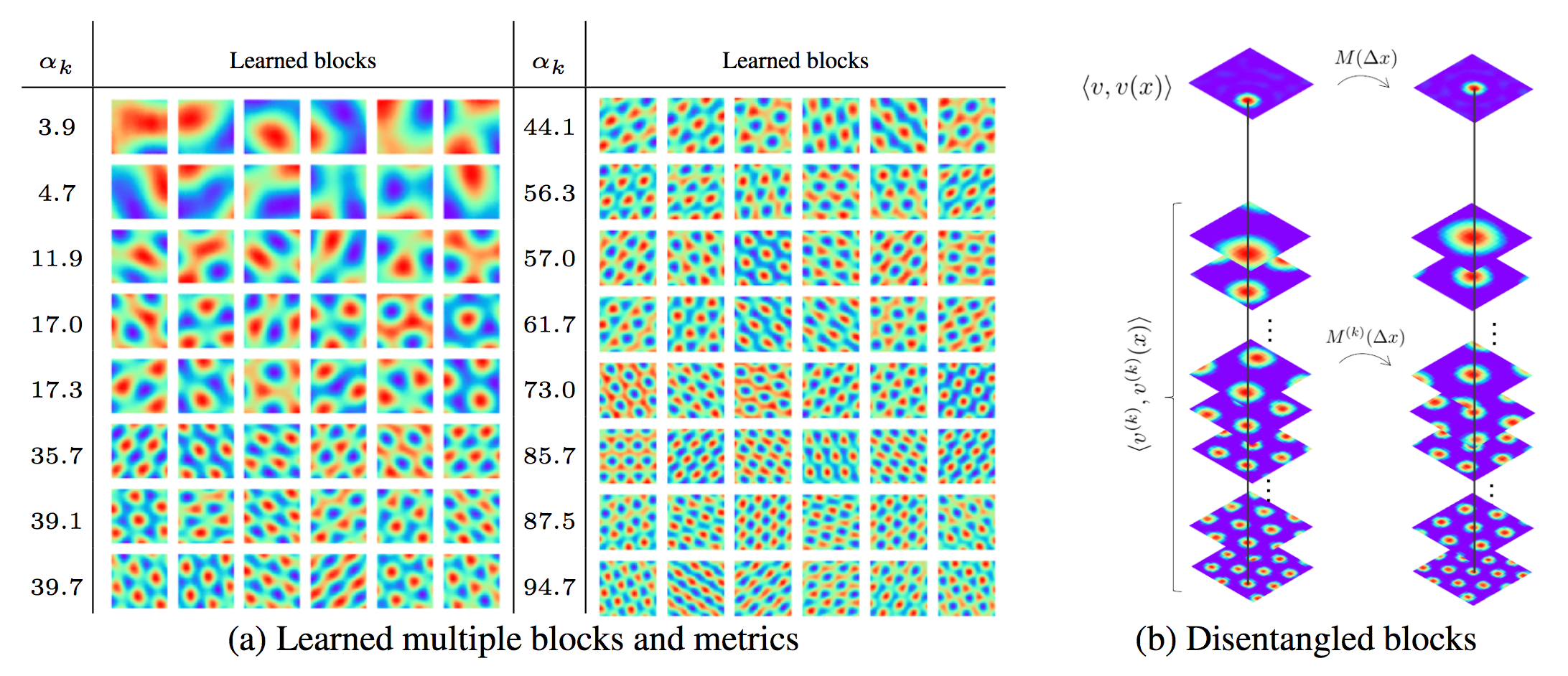Figure 4. (a) Response maps of learned units of the vector representation and learned scaling parameters αk. Block size equals 6 and each row shows the units belonging to the same block. (b) Illustration of block-wise activities of hidden units (where the activities are rectified to be positive).

### Experiment 3: Path Integral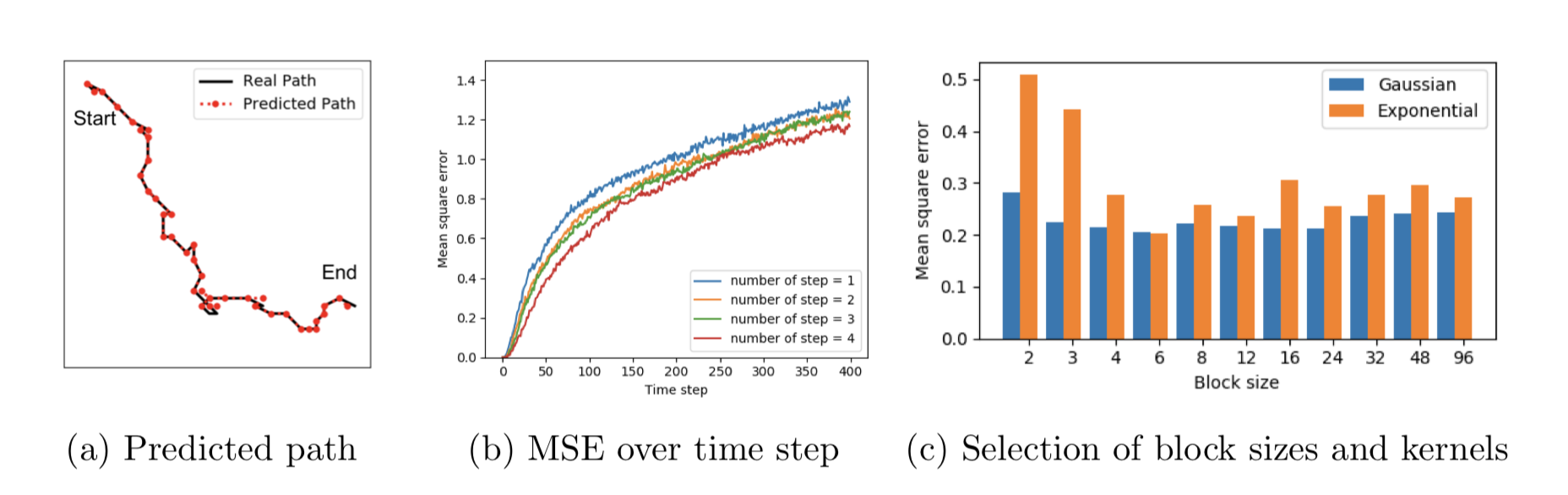Figure 5. (a) Path integral prediction. The black line depicts the real path while red dotted line is the predicted path by the learned model. (b) Mean square error over time step. The error is average over 1,000 episodes. The curves correspond to different number of steps used in the multi-step motion loss. (c) Mean square error performed by models with different block sizes and different kernel types. Error is measured by number of grids.

### Experiment 4: Path Planning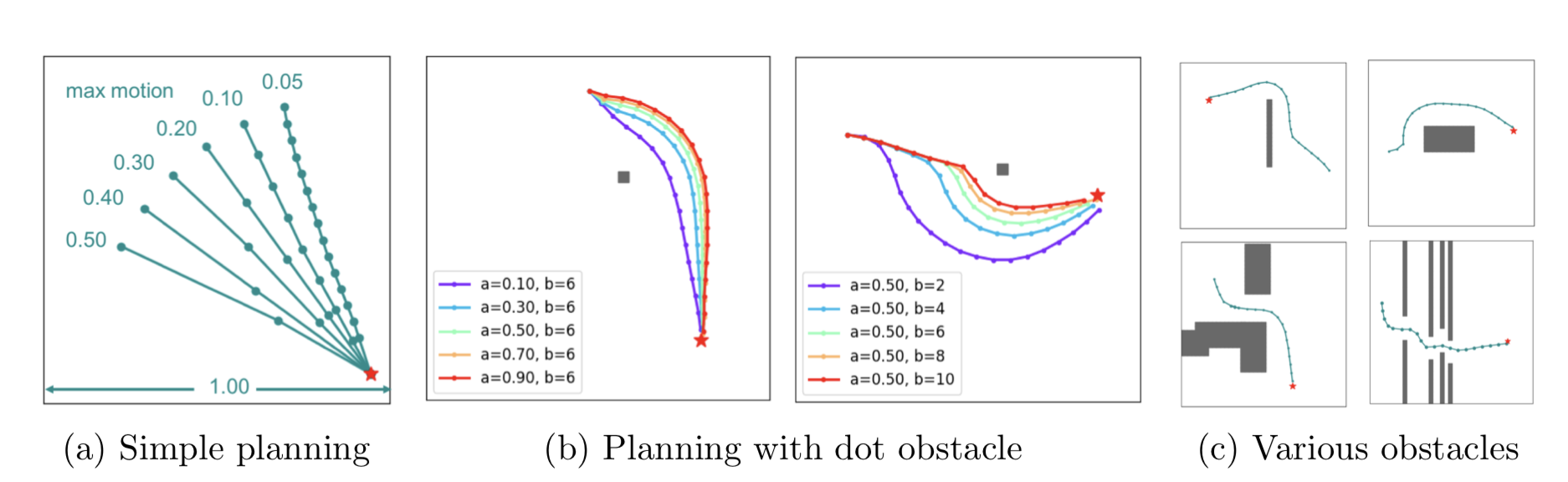Figure 6. (a) Planning examples with different motion ranges. Red star represents the destination y and green dots represent the planned position {x0 + Σti=1Δxi}. (b) Planning examples with a dot obstacle. Left figure shows the effect of changing scaling parameter a, while right figure shows the effect of changing annealing parameter b. (c) Planning example with obstacles mimicking walls, large objects and simple mazes.

### Experiment 5: Quantitative analysis of spatial activity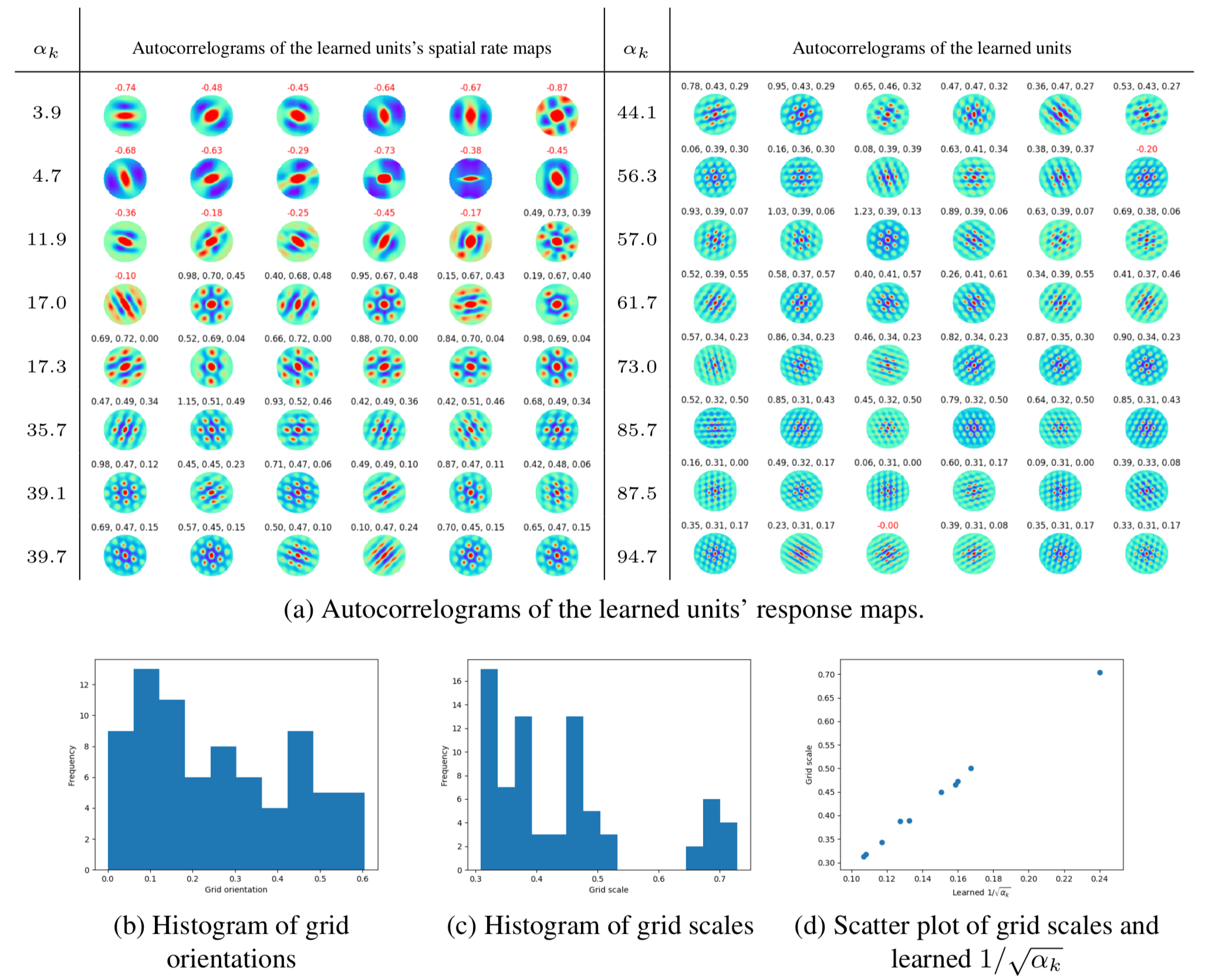Figure 7. (a) Autocorrelograms of the learned units’ response maps. Gridness scores are calculated based on the autocorrelograms. A unit is classified as a grid cell if the gridness score is larger than 0. The gridness score is shown in red color if a unit fails to be classified as a grid cell. For those units that are classified as grid cells, gridness score, scale and orientation are listed sequentially in black color. Orientation is computed using a camera-fixed reference line (0o) and in counterclockwise direction. (b) Histogram of grid orientations. (c) Histogram of grid scales. (d) Scatter plot of averaged grid scales within each block versus the corresponding learned 1/ √αk.

### Experiment 6: Error correction

Table 1. Error correction results on the vector representation. The performance of path integral is measured by mean square error between predicted locations and ground truth locations; while for path planning, the performance is measured by success rate. Experiments are conducted using several noise levels: Gaussian noise with different standard deviations in terms of the reference standard deviation s and dropout mask with different percentages. DE means implementing decoding-encoding process when performing the tasks.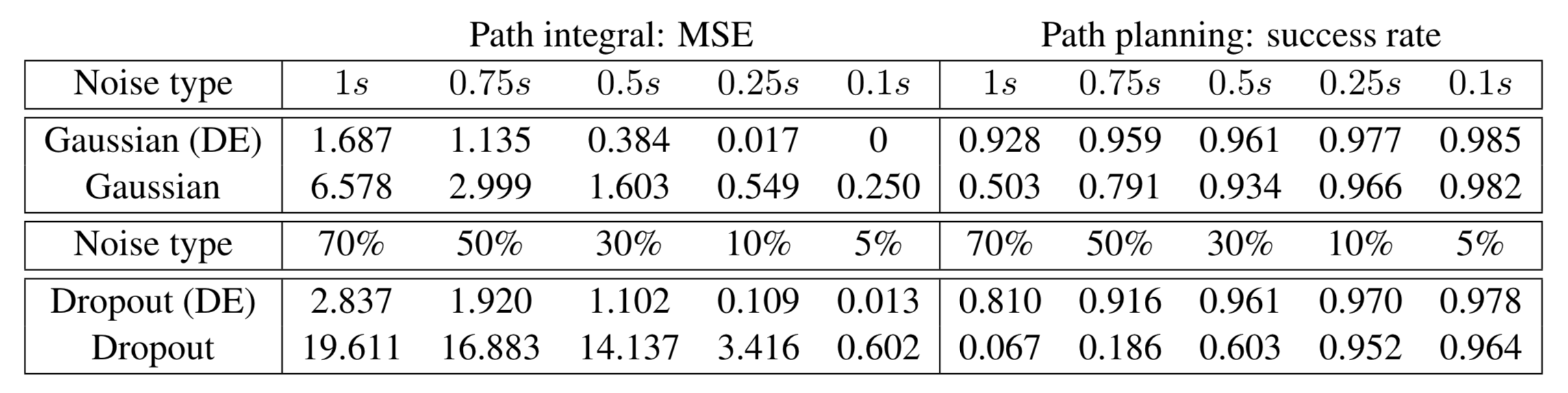### Acknowledgment

We thank the three reviewers for their insightful comments and suggestions. Part of the work was done while Ruiqi Gao was an intern at Hikvision Research Institute during the summer of 2018. She thanks Director Jane Chen for her help and guidance. We also thank Jiayu Wu for her help with experiments and Zilong Zheng for his help with visualization. The work is supported by DARPA XAI project N66001-17-2-4029; ARO project W911NF1810296; ONR MURI project N00014-16-1-2007; and a Hikvision gift to UCLA. We gratefully acknowledge the support of NVIDIA Corporation with the donation of the Titan Xp GPU used for this research.

### Related Reference

 Hafting, Torkel, et al. "Microstructure of a spatial map in the entorhinal cortex." Nature 436.7052 (2005): 801.

 Fyhn, Marianne, et al. "Grid cells in mice." Hippocampus 18.12 (2008): 1230-1238.

 Banino, Andrea, et al. "Vector-based navigation using grid-like representations in artificial agents." Nature 557.7705 (2018): 429.

 Cueva, Christopher J., and Xue-Xin Wei. "Emergence of grid-like representations by training recurrent neural networks to perform spatial localization." arXiv preprint arXiv:1803.07770 (2018).